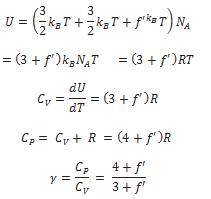# Specific Heat Capacity

1. Monatomic Gases
2. Diatomic Gases
3. Polyatomic Gases

## Specific Heats of Monatomic Gases:

The molecule of a monatomic gas has only three translational degrees of freedom.

Thus, the average energy of a molecule at temperature T is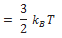The total internal energy of a mole of such a gas is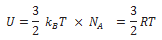The molar specific heat at constant volume, Cv is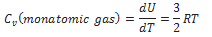For an ideal gas,where Cp is the molar specific heat at constant pressure. Thus,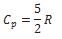The ratio of specific heats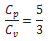## Specific Heats of Diatomic Gases:

A diatomic molecule treated as a rigid rotator like a dumbbell has 5 degrees of freedom: 3 translational and 2 rotational. Using the law of equipartition of energy, the total internal energy of a mole of such a gas is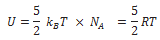The molar specific heats are then given by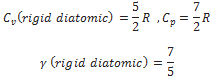If the diatomic molecule is not rigid but has, in addition, a vibration mode## Specific Heats of Polyatomic Gases:

A polyatomic molecule has 3 translational, 3 rotational degrees of freedom and a certain number (f) of vibration modes. According to the law of equipartition of energy, it is easily seen that one mole of such a gas has